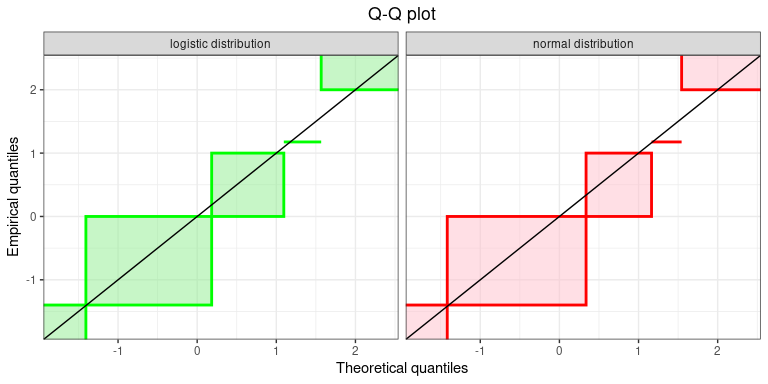1 Questions regarding distributions

1.1 How do I know the root name of a distribution?

The root name of a probability distribution is the name which is used the d, p, q, r functions. For base R distributions, the root names are given in R-intro : https://cran.r-project.org/doc/manuals/R-intro.html#Probability-distributions. For example, you must use "pois" for the Poisson distribution and not "poisson".

1.2 How do I find “non standard” distributions?

For non-standard distributions, you can either find a package implementing them or define by yourself. A comprehensive list of non-standard distributions is given in the Distributions task view https://CRAN.R-project.org/view=Distributions. Here are some two examples of user-defined distributions. A third example (shifted exponential) is given in FAQ 3.5.4.

• The Gumbel distribution
• The zero-modified geometric distribution

1.3 How do I set (or find) initial values for non standard distributions?

As documented, we provide initial values for the following distributions: "norm", "lnorm", "exp", "pois", "cauchy", "gamma", "logis", "nbinom", "geom", "beta", "weibull" from the stats package; "invgamma", "llogis", "invweibull", "pareto1", "pareto", "lgamma", "trgamma", "invtrgamma" from the actuar package.

Look first at statistics and probability books such as

• different volumes of N. L. Johnson, S. Kotz and N. Balakrishnan books, e.g. Continuous Univariate Distributions, Vol. 1,
• Thesaurus of univariate discrete probability distributions by G. Wimmer and G. Altmann.
• Statistical Distributions by M. Evans, N. Hastings, B. Peacock.
• Distributional Analysis with L-moment Statistics using the R Environment for Statistical Computing by W. Asquith.

If not available, find initial values by equalling theoretical and empirical quartiles. The graphical function plotdist() and plotdistcens() can also be used to assess the suitability of starting values : by an iterative manual process you can move parameter values so as to obtain a distribution that roughly fits the data and take these parameter values as starting values for the real fit. You may also consider the prefit() function to find initial values especially in case where parameters are constrained.

1.4 Is it possible to fit a distribution with at least 3 parameters?

Yes, an example with the Burr distribution is detailed in the JSS paper. We reproduce it very quickly here.

## Fitting of the distribution ' burr ' by maximum likelihood
## Parameters :
##        estimate Std. Error
## shape1    0.206     0.0561
## shape2    1.540     0.3188
## rate      1.497     0.4683
## Loglikelihood:  -520   AIC:  1046   BIC:  1054
## Correlation matrix:
##        shape1 shape2   rate
## shape1  1.000 -0.900 -0.727
## shape2 -0.900  1.000  0.588
## rate   -0.727  0.588  1.000

1.5 Why there are differences between MLE and MME for the lognormal distribution?

We recall that the lognormal distribution function is given by $F_X(x) = \Phi\left(\frac{\log(x)-\mu}{\sigma} \right),$ where $$\Phi$$ denotes the distribution function of the standard normal distribution. We know that $$E(X) = \exp\left( \mu+\frac{1}{2} \sigma^2 \right)$$ and $$Var(X) = \exp\left( 2\mu+\sigma^2\right) (e^{\sigma^2} -1)$$. The MME is obtained by inverting the previous formulas, whereas the MLE has the following explicit solution $\hat\mu_{MLE} = \frac{1}{n}\sum_{i=1}^n \log(x_i),~~ \hat\sigma^2_{MLE} = \frac{1}{n}\sum_{i=1}^n (\log(x_i) - \hat\mu_{MLE})^2.$ Let us fit a sample by MLE and MME. The fit looks particularly good in both cases.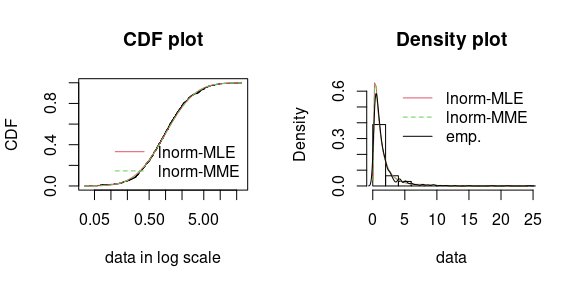Let us compare the theoretical moments (mean and variance) given the fitted values ($$\hat\mu,\hat\sigma$$), that is $E(X) = \exp\left( \hat\mu+\frac{1}{2} \hat\sigma^2 \right), Var(X) = \exp\left( 2\hat\mu+\hat\sigma^2\right) (e^{\hat\sigma^2} -1).$

## E(X) by MME E(X) by MLE   empirical
##        1.61        1.60        1.61
## Var(X) by MME Var(X) by MLE     empirical
##          4.30          4.36          4.30

From a MLE point of view, a lognormal sample $$x_1,\dots,x_n$$ is equivalent to handle a normal sample $$\log(x_1),\dots,\log(x_n)$$. However, it is well know by the Jensen inequality that $$E(X) = E(\exp(\log(X))) \geq \exp(E(\log(X)))$$ implying the MME estimates provides better moment estimates than with MLE.

1.6 Can I fit a distribution with positive support when data contains negative values?

The answer is no: you cannot fit a distribution with positive support (say gamma distribution) when data contains negative values.

## Error in computing default starting values.
## Error in manageparam(start.arg = start, fix.arg = fix.arg, obs = data,  :
##   Error in start.arg.default(obs, distname) :
##   values must be positive to fit an exponential  distribution
##  "Error in manageparam(start.arg = start, fix.arg = fix.arg, obs = data,  : \n  Error in start.arg.default(obs, distname) : \n  values must be positive to fit an exponential  distribution\n\n"
## attr(,"class")
##  "try-error"
## attr(,"condition")
## <simpleError in manageparam(start.arg = start, fix.arg = fix.arg, obs = data,     distname = distname): Error in start.arg.default(obs, distname) :
##   values must be positive to fit an exponential  distribution
## >

It is irrelevant to do such fit. If you really need to use that distribution, you have two options: either to remove negative values (not recommended) or to shift the data.

## Fitting of the distribution ' exp ' by maximum likelihood
## Parameters:
##      estimate Std. Error
## rate     1.06      0.107
## Fitting of the distribution ' exp ' by maximum likelihood
## Parameters:
##      estimate Std. Error
## rate    0.914     0.0914

1.7 Can I fit a finite-support distribution when data is outside that support?

The answer is no: you cannot fit a distribution with finite-support (say beta distribution) when data is outside $$[0,1]$$.

## Error in computing default starting values.
## Error in manageparam(start.arg = start, fix.arg = fix.arg, obs = data,  :
##   Error in start.arg.default(obs, distname) :
##   values must be in [0-1] to fit a beta distribution
##  "Error in manageparam(start.arg = start, fix.arg = fix.arg, obs = data,  : \n  Error in start.arg.default(obs, distname) : \n  values must be in [0-1] to fit a beta distribution\n\n"
## attr(,"class")
##  "try-error"
## attr(,"condition")
## <simpleError in manageparam(start.arg = start, fix.arg = fix.arg, obs = data,     distname = distname): Error in start.arg.default(obs, distname) :
##   values must be in [0-1] to fit a beta distribution
## >

It is irrelevant to do such a fit. If you really need to use that distribution, you have two ways to tackle this issue: either to remove impossible values (not recommended) or to shift/scale the data.

## Fitting of the distribution ' beta ' by maximum likelihood
## Parameters:
##        estimate Std. Error
## shape1     2.08      0.288
## shape2     2.50      0.352
## Fitting of the distribution ' beta ' by maximum likelihood
## Parameters:
##        estimate Std. Error
## shape1     1.77      0.236
## shape2     2.17      0.296

1.8 Can I fit truncated distributions?

The answer is yes: but the fitting procedure must be carried out carefully. Let $$X$$ be the original untruncated random variable. The truncated variable is the conditionnal random variable $$Y = X ~\vert~ l< X <u$$ with $$l<u$$ the lower and upper bounds. The cdf of $$Y$$ is $$F_Y(y)=\frac{F_X(x) - F_X(l)}{F_X(u)-F_X(l)}$$. There is a density (w.r.t. the Lebesgues measure) given by $f_Y(y) = \left\{\begin{array}{ll} \frac{f_X(x)}{F_X(u)-F_X(l)} & \text{if } l < x < u \\ 0 & \text{otherwise }\\ \end{array}\right.$

## Goodness-of-fit statistics
##                              1-mle-texp 2-mle-texp
## Kolmogorov-Smirnov statistic     0.0952      0.084
## Cramer-von Mises statistic       0.1343      0.104
## Anderson-Darling statistic          Inf      1.045
##
## Goodness-of-fit criteria
##                                1-mle-texp 2-mle-texp
## Akaike's Information Criterion        127        132
## Bayesian Information Criterion        130        135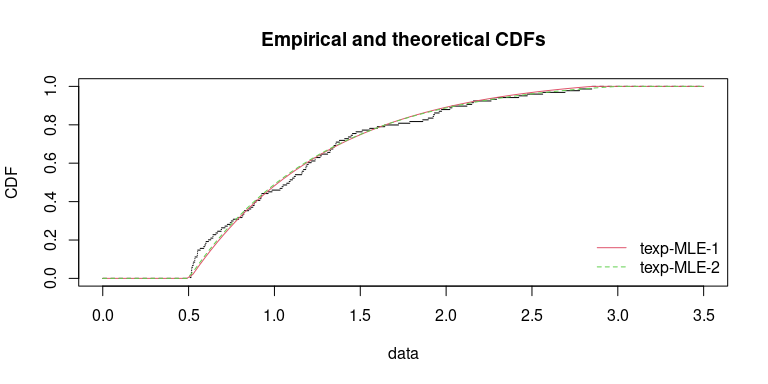1.9 Can I fit truncated inflated distributions?

The answer is yes: but the fitting procedure must be carried out carefully. Let $$X$$ be the original untruncated random variable. The truncated variable is $$Y = \max(\min(X, u), l)$$ with $$l<u$$ the lower and upper bounds. The cdf of $$Y$$ is $$F_Y(y)=F_X(y)1_{u>y>l} + 1_{y>u}$$. There is no density (w.r.t. the Lebesgues measure) since there are two probability masses $$P(Y=l)= P(X\leq l)>0$$ and $$P(Y=u)=P(X>u)>0$$. However, the density function with respect to the measure $$m(x)= \delta_l(x)+\delta_u(x)+\lambda(x)$$ is $f_Y(y) = \left\{\begin{array}{ll} F_X(l) & \text{if } y=l \\ f_X(y) & \text{if } l<y<u \\ 1-F_X(u) & \text{if } y=u \\ \end{array}\right.$ Let $$\theta$$ be the parameter of the untruncated distribution. Since the likelihood can be factorized, the maximization can be done separately $L(l, \theta, u) = 1_{\forall i, l\leq y_i\leq u} \prod_{i=1, y_i=l}^n F_X(l, \theta) \times \prod_{i=1,l<y_i<u}^n f_X(y_i, \theta) \times \prod_{i=1,y_i=u}^n (1-F_X(u, \theta)),$ Furthermore, using $$\forall i, l\leq y_i\leq u\Leftrightarrow l\leq \min_i y_i\leq \max_i y_i\leq u$$, the likelihood is zero for $$l>\min_i y_i$$ or $$u<\max_i y_i$$ and increasing with respect to $$l$$ in $$]-\infty, \min_i y_i]$$ and decreasing with respect to $$u$$ in $$[\max_i y_i,+\infty[$$. So the maximum of $$L$$ is reached at $$l=\min_i y_i$$ and $$u=\max_i y_i$$. The MLE of $$\theta$$ is then obtained by maximizing the log-likelihood $$\log(L(l, \theta, u))$$ with $$u=\max_i Y_i$$ and $$l=\min_i Y_i$$.

Let us illustrate truncated distribution with the truncated exponential distribution. The log-likelihood is particularly bad-shaped.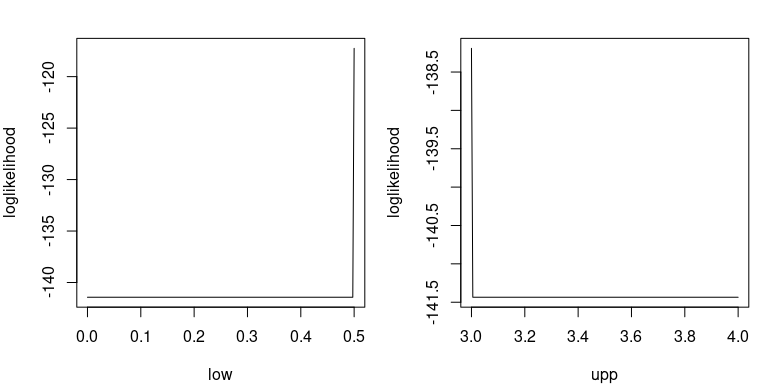The first method directly maximizes the log-likelihood $$L(l, \theta, u)$$; the second method maximizes the log-likelihood $$L(\theta)$$ assuming that $$u=\hat u$$ and $$l=\hat l$$ are known. Inside $$[0.5,3]$$, the CDF are correctly estimated in both methods but the first method does not succeed to estimate the true value of the bounds $$l,u$$.

## Fitting of the distribution ' tiexp ' by maximum likelihood
## Parameters:
##      estimate Std. Error
## rate    0.949         NA
## low    -0.502         NA
## upp    23.072         NA
## Fitting of the distribution ' tiexp ' by maximum likelihood
## Parameters:
##      estimate Std. Error
## rate    0.947     0.0982
## Fixed parameters:
##     value
## low   0.5
## upp   3.0
## Goodness-of-fit statistics
##                              1-mle-tiexp 2-mle-tiexp
## Kolmogorov-Smirnov statistic       0.378       0.377
## Cramer-von Mises statistic         1.890       1.882
## Anderson-Darling statistic        10.222      10.193
##
## Goodness-of-fit criteria
##                                1-mle-tiexp 2-mle-tiexp
## Akaike's Information Criterion         216         162
## Bayesian Information Criterion         224         165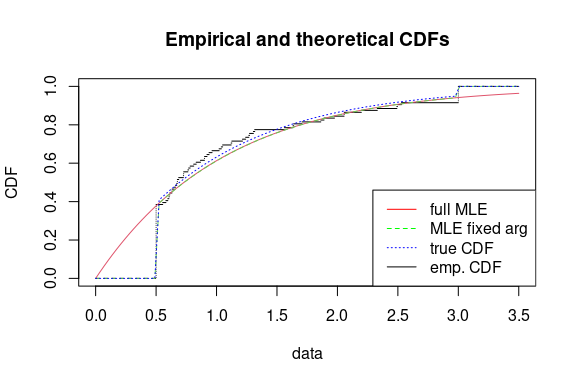1.10 Can I fit a uniform distribution?

The uniform distribution $$\mathcal U(a,b)$$ has only support parameters since the density does not have a scale or a shape parameter $$f_U(u) = \frac{1}{b-a}1_{[a,b]}(u)$$. For this distribution, we should not maximize the log-likelihood but only the likelihood. Let $$(x_i)_i$$ be i.i.d. observations from $$\mathcal U(a,b)$$ distribution. The likelihood is $L(a,b) = \prod_{i=1}^n \frac{1}{b-a} 1_{[a,b]}(x_i) = 1_{a\leq x_i \leq b, i=1,\dots,n} \frac{1}{b-a}^n = 1_{a\leq \min_i x_i} 1_{\max_i x_i \leq b} \frac{1}{b-a}^n$ Hence $$a\mapsto L(a,b)$$ for any fixed $$b\in]\max_i x_i, +\infty[$$ is increasing on $$]-\infty, \min_i x_i]$$, similarly $$b\mapsto L(a,b)$$ is decreasing for any fixed $$a$$. This leads to $$\min_i x_i$$ and $$\max_i x_i$$ to be the MLE of the uniform distribution.

We should notice that the likelihood function $$L$$ is defined on $$\mathbb R^2$$ yet it cancels outside $$S=]-\infty, \min_i x_i]\times]\max_i x_i, +\infty[$$. Hence, the log-likelihood is undefined outside $$S$$, which is an issue when maximizing the log-likelihood.

For these reasons, fitdist(data, dist="unif", method="mle") uses the explicit form of the MLE for this distribution. Here is an example below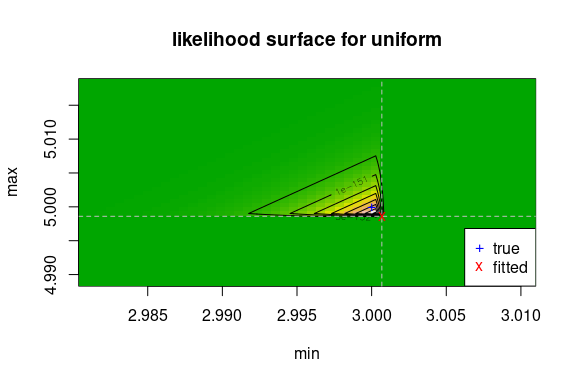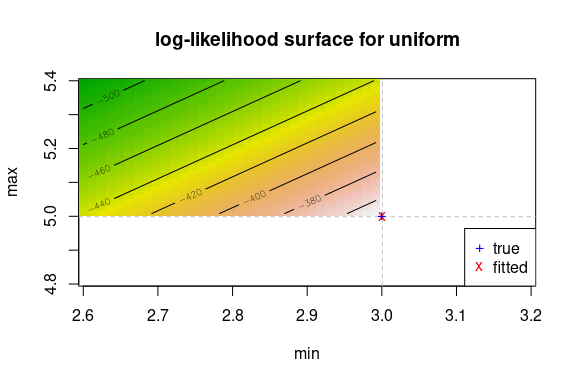Maximizing the log-likelihood is harder and can be done by defining a new density function. Appropriate starting values and parameters bound must be supplied. Using the closed-form expression (as in fitdist()) or maximizing the log-likelihood (with unif2) lead to very similar results.

##  -346.0539 -346.0540
##         [,1]     [,2]
## min 3.000684 3.000683
## max 4.998606 4.998606

1.11 Can I fit a beta distribution with the same shape parameter?

Yes, you can wrap the density function of the beta distribution so that there is a only one shape parameter. Here is an example of a concave density.

## Fitting of the distribution ' beta2 ' by maximum likelihood
## Parameters:
##       estimate Std. Error
## shape     3.24      0.135

Another example with a U-shaped density.

## Fitting of the distribution ' beta2 ' by maximum likelihood
## Parameters:
##       estimate Std. Error
## shape    0.295    0.00986

1.12 How to estimate support parameter? the case of the four-parameter beta

Let us consider the four-parameter beta distribution, also known as the PERT distribution, defined by the following density for $$x\in [a,c]$$ $$f_X(x) = (x-a)^{\alpha-1} (c-x)^{\beta-1}/C_N$$ with $$C_N$$ a normalizing constant and $$\alpha=1+d(b-a)/(c-a)$$, $$\beta=1+d(c-b)/(c-a)$$. $$a,c$$ are support parameters, $$b\in]a,c[$$ is the mode and $$d$$ the shape parameter.

As for uniform distribution, one can show that the MLE of $$a$$ and $$c$$ are respectively the sample minimum and maximum. The code below illustrates the strategy using partial closed formula with fix.arg and the full numerical search of MLE. NB: on small sample size, the latter has generally better goodness-of-fit statistics; a small positive number is added or subtracted when fixing the support parameters $$a$$ and $$c$$ to sample minimum and maximum.

## Warning in checkparamlist(arg_startfix$start.arg, arg_startfix$fix.arg, : Some
## parameter names have no starting/fixed value but have a default value: mean.
## Warning in checkparamlist(arg_startfix$start.arg, arg_startfix$fix.arg, : Some
## parameter names have no starting/fixed value but have a default value: mean.
## Goodness-of-fit statistics
##                              1-mle-pert 2-mle-pert
## Kolmogorov-Smirnov statistic     0.0452     0.0584
## Cramer-von Mises statistic       0.0823     0.1834
## Anderson-Darling statistic       0.5325     1.2776
##
## Goodness-of-fit criteria
##                                1-mle-pert 2-mle-pert
## Akaike's Information Criterion        268        265
## Bayesian Information Criterion        281        272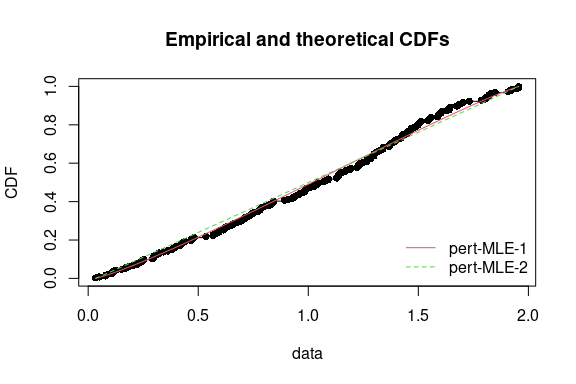##       [,1]       [,2]
## min   0.03106317 0.03395487
## mode  1.120283   1.956149
## max   1.9595     1.956234
## shape 0.3056077  0.008646087

2 Questions regarding goodness-of-fit tests and statistics

2.1 Where can we find the results of goodness-of-fit tests ?

Results of goodness-of-fit tests are not printed but are given in the object returned by gofstat() and you can have access to them as described in the example below. Nevertheless, p-values are not given for every test. For Anderson-Darling (ad), Cramer von Mises (cvm) and Kolomogorov (ks), only the decision (rejection of H0 or not) is given, when available (see FAQ 2.3 for more details).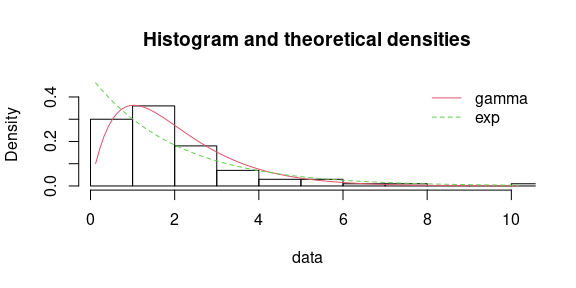##    gamma      exp
## 1.89e-01 7.73e-05
##           obscounts theo gamma theo exp
## <= 0.5483         9      10.06    23.66
## <= 0.8122         9       8.82     9.30
## <= 0.9592         9       5.27     4.68
## <= 1.368          9      14.63    11.37
## <= 1.523          9       5.24     3.74
## <= 1.701          9       5.73     3.97
## <= 1.94           9       7.09     4.82
## <= 2.381          9      11.08     7.50
## <= 2.842          9       9.00     6.29
## <= 3.801          9      11.93     9.28
## > 3.801          10      11.16    15.40
##          gamma            exp
## "not rejected"     "rejected"
##          gamma            exp
## "not rejected"     "rejected"
##          gamma            exp
## "not rejected"     "rejected"

2.2 Is it reasonable to use goodness-of-fit tests to validate the fit of a distribution ?

In the first versions of fitdistrplus, when they were available, the results of the GOF tests (AD, KS, CvM) were automatically printed. We decided to suppress this automatic printing when we realized that some users had some difficulties to interpret the results of those tests and sometimes misused them.

Goodness-of-fit tests often appear as objective tools to decide wether a fitted distribution well describes a data set. But they are not ! It would not be reasonable at all to reject a distribution just because a goodness-of-fit test rejects it (see FAQ 2.2.1). And it would not be reasonable at all any more to validate a distribution because goodness-of-fit tests do not reject it (see FAQ 2.2.2).

A fitted distribution should be evaluated using graphical methods (goodness-of-fit graphs automatically provided in our package by plotting the result of the fit (output of fitdist() or fitdistcens() and the complementary graphs that help to compare different fits - see ?graphcomp). We really think it is the most appropriate way to evaluate the adequacy of a fit and we are not the only ones to recommend it. You can find the same type of recommendations in reference books :

• Probabilistic techniques in exposure assessment - a handbook dealing with variability and uncertainty in models and inputs by A.C. Cullen and H.C. Frey.
• Application of uncertainty analysis to ecological risks of pesticides by W.J. Warren-Hicks and A. Hart.
• Statistical inference by G. Casella and R.L. Berger
• Loss models: from data to decision by S.A. Klugman and H.H. Panjer and G.E. Willmot

Moreover, the selection of a distribution should also be driven by knowledge of underlying processes when available. For example when a variable cannot be negative, one would be very cautious while fitting a normal distribution, that potentially gives negative values, even if the observed data of this variable seem well fitted by a normal distribution.

2.2.1 Should I reject a distribution because a goodness-of-fit test rejects it ?

No it would not be reasonable at all to reject a distribution just because a goodness-of-fit test rejects it, especially in the case of big samples. In the real life, as soon as you have a sufficient amount of data, you will reject the fitted distribution. We know that a model cannot perfectly describe real data, and generally the true question is to find the better distribution among a pool of simple parametric distributions to describe the data, so to compare different models (see FAQ 2.4 and 2.5 for corresponding questions).

To illustre this point let us comment the example presented below. We drew two samples from the same Poisson distribution with a mean parameter equal to 100. In many applications, for this value of its parameter, the Poisson distribution would be considered to be well approximated by a normal distribution. Testing the fit (here using a Kolmogorov-Smirnov test ) of the normal distribution on a sample of 100 observations would not reject the normal fit, while testing it on a sample of 10000 observations would reject it, while both samples come from the same distribution.

##     1-mle-norm
## "not rejected"
## 1-mle-norm
## "rejected"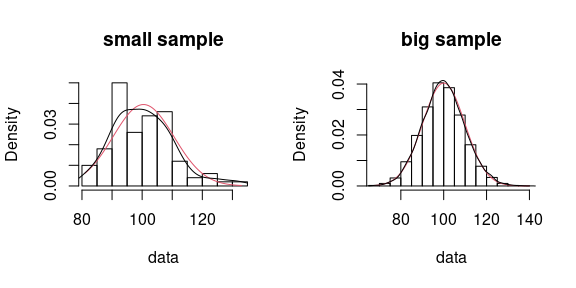2.2.2 Should I accept a distribution because goodness-of-fit tests do not reject it ?

No, it would not be reasonable at all to validate a distribution because goodness-of-fit tests do not reject it. Like all the other hypothesis tests, goodness-of-fit tests lack of statistical power when the sample size is not so high. And the different goodness-of-fit tests are not equally sensitive to different types of deviation between empirical and fitted distributions. For example the Kolmogorov-Smirnov test is sensitive when distributions differ in a global fashion near the centre of the distribution. The Anderson-Darling test will be more sensitive when distributions differ in their tails, and the Cramer von Mises will be more sensitive when there are small but repetitive differences between empirical and theoretical distribution functions.

The sensitivity of a chi square test will depend on the definition of classes, and even if we propose a default definition of classes when the user does not provide classes, this choice is not obvious and could impact the results of the test. This test is more appropriate when data are discrete, even if they are modelled by a continuous distribution, as in the following example. Two samples of respective sizes 500 and 50 are drawn from a Poisson distribution of mean parameter equal to 1 (not a sufficiently high value to consider that the Poisson distribution could be approximated by a normal one). Using a Kolmogorov-Smirnov test, for the small sample the normal fit is rejected only for the bigger sample. It is not rejected with the smaller sample even if the fit could be rejected after a simple visual confrontation of the distributions. In that particular case, the chi square test with classes defined by default would have rejected te normal fit for both samples.

## 1-mle-norm
## "rejected"
##     1-mle-norm
## "not rejected"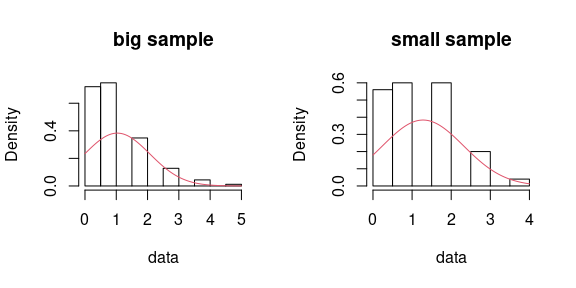##      obscounts theocounts
## <= 0     180.0       80.3
## <= 1     187.0      163.5
## <= 2      87.0      168.1
## <= 3      32.0       73.4
## > 3       14.0       14.7
##  7.11e-42
##      obscounts theocounts
## <= 0     14.00       5.46
## <= 1     15.00      14.23
## <= 2     15.00      18.09
## > 2       6.00      12.22
##  3.57e-05

2.3 Why all goodness-of-fit tests are not available for every distribution ?

The Chi-squared test is available for any distribution but one must be conscious that its result depends on the definition of cells in which observed data are grouped, and a correct definition is not possible with a too small sample.

Concerning the Kolmogorov-Smirnov test, it is proposed for any continuous distribution, but with a critical value corresponding to the comparison of the empirical distribution to a fully specified distribution. As the distribution is not fully known for a fitted distribution, the result of this test is subject to caution, but there is no general asymptotic theory for the Kolmogorov-Smirnov statistics in case of a fitted distribution. Nevertheless, one can use Monte Carlo methods to conduct Kolmgorov-Smirnov goodness-of-fit tests in cases when the sample is used to estimate model parameters. Such a method is implemented in the R package KScorrect for a variety of continuous distributions.

Such an asymptotic theory was proposed for quadratic statistics for some distributions (Anderson-Darling, Cramer von Mises). The reference book we used on this subject (Tests based on edf statistics by Stephens MA in Goodness-of-fit techniques by D’Agostino RB and Stephens MA) proposes critical values of those statistics for a some classical distributions (exponential, gamma, Weibull, logistic, Cauchy, normal and lognormal). But the asymptotic theory about these statistics also depends on the way the parameters are estimated. And as they were not estimated by maximum likelihood for Cauchy, normal and lognormal distributions in the results reported by Stephens, we only propose the results of the Anderson-Darling and Cramer von Mises using those results for exponential, gamma, Weibull, logistic distributions.

The user can refer to the cited books and use the proposed formula to estimate the parameters of Cauchy, normal and lognormal distributions and apply the tests using critical values given in the book. R packages goftest and ADGofTest could also be explored by users who would like to apply Anderson-Darling and Cramer von Mises tests on other distributions. But at this time we are not sure that the case where parameters are unknown (estimated by maximum likelihood) is tackled in those two packages.

Concerning the development of our package, rather than develoing further more goodness-of-fit tests we made the choice to develop graphical tools to help to appreciate the quality of a fit and to compare the fits of different distributions on a same data set (see FAQ 2.2 for argumentation).

2.4 How can we use goodness-of-fit statistics to compare the fit of different distributions on a same data set ?

Goodness-of-fit statistics based on the empirical distribution function (Kolmogorov-Smirnov, Anderson-Darling and Cramer von Mises) may be used to measure a distance between the fitted distribution and the empirical distribution. So if one wants to compare the fit of various distributions on the same data set, the smaller are those statistics the better. The Kolmogorov-Smirnov statistics will be sensitive when distributions differ in a global fashion near the centre of the distribution while the Anderson-Darling statistics will be more sensitive when distributions differ in their tails, and the Cramer von Mises statistics will be more sensitive when there are small but repetitive differences between empirical and theoretical distribution functions.

But as mentioned in the main vignette of our package, the use of the Anderson-Darling to compare the fit of different distributions is subject to caution due to the the weighting of the quadratic distance between fitted and empirical distribution functions that depends on the parametric distribution. Moreover, statistics based on the empirical distribution function do not penalize distributions with a greater number of parameters and as those are generally more flexible, this could induce over-fitting.

Goodness-fo-fit statistics based on information criteria (AIC, BIC) correspond to deviance penalized by the complexity of the model (the number of parameters of the distribution), and so the smaller the better. As more generic statistics, they are not adapted to focus on a part of the fitted distribution, but they take into account the complexity of the distribution and thus could help to prevent overfitting.

2.5 Can we use a test to compare the fit of two distributions on a same data set ?

In our package we did not implement such a test but for two nested distributions (when one is a special case of the other one, e.g. exponential and gamma distributions) a likelihood ratio test can be easily implemented using the loglikelihood provided by fitdist or fitdistcens. Denoting $$L$$ the maximum likelihood obtained with the complete distribution and $$L_0$$ the one obtained with the simplified distribution, when the sample size increases, $$- 2 ln(\frac{L_0}{L}) = 2 ln(L) - 2 ln(L_0)$$ tends to a Chi squared distribution degrees of freedom equal to the difference on the numbers of parameters characterizing the two nested distributions. You will find below an example of such a test.

## Fitting of the distribution ' gamma ' by maximum likelihood
## Parameters:
##       estimate Std. Error
## shape    2.025      0.266
## rate     0.997      0.149
## Fitting of the distribution ' exp ' by maximum likelihood
## Parameters:
##      estimate Std. Error
## rate    0.492     0.0492
##  23.9
##  3.84
##  TRUE

Such a test can also be used for fits on censored data.

2.6 Can we get goodness-of-fit statistics for a fit on censored data ?

Function gofstat is not yet proposed in our package for fits on censored data but to develop one is among one of our objectives in the future. Published works on goodness-of-fit statistics based on the empirical distribution function for censored data generally focused on data containing only one type of censoring (e.g. right censored data in survival data). Build such statistics in the general case, with data containing in the same time (right, left and interval censoring), remains tricky.

Nevertheless, it is possible for any type of censored data, to use information criteria (AIC and BIC given in the summary of an object of class fitdistcens) to compare the fits of various distributions to a same data set.

3 Questions regarding optimization procedures

3.1 How to choose optimization method?

If you want to perform optimization without bounds, optim() is used. You can try the derivative-free method Nelder-Mead and the Hessian-free method BFGS. If you want to perform optimization with bounds, only two methods are available without providing the gradient of the objective function: Nelder-Mead via constrOptim() and bounded BFGS via optim(). In both cases, see the help of mledist() and the vignette on optimization algorithms.

3.2 The optimization algorithm stops with error code 100. What shall I do?

First, add traces by adding control=list(trace=1, REPORT=1). Second, try to set bounds for parameters. Third, find better starting values (see FAQ 1.3).

3.3 Why distribution with a log argument may converge better?

Say, we study the shifted lognormal distribution defined by the following density $f(x) = \frac{1}{x \sigma \sqrt{2 \pi}} \exp\left(- \frac{(\ln (x+\delta)- \mu)^2}{2\sigma^2}\right)$ for $$x>-\delta$$ where $$\mu$$ is a location parameter, $$\sigma$$ a scale parameter and $$\delta$$ a boundary parameter. Let us fit this distribution on the dataset y by MLE. We define two functions for the densities with and without a log argument.

We now optimize the minus log-likelihood.

## Fitting of the distribution ' shiftlnorm ' by maximum likelihood
## Parameters :
##       estimate Std. Error
## mean    -1.386    0.02401
## sigma    0.071    0.00192
## shift    0.248    0.00598
## Loglikelihood:  8299   AIC:  -16591   BIC:  -16573
## Correlation matrix:
##         mean  sigma  shift
## mean   1.000 -0.885  0.999
## sigma -0.885  1.000 -0.886
## shift  0.999 -0.886  1.000

If we don’t use the log argument, the algorithms stalls.

## <simpleError in optim(par = vstart, fn = fnobj, fix.arg = fix.arg, obs = data,     gr = gradient, ddistnam = ddistname, hessian = TRUE, method = meth,     lower = lower, upper = upper, ...): différences finies ayant des valeurs infinies >
## Error in fitdist(y, "shiftlnorm_no", start = start, optim.method = "BFGS") :
##   the function mle failed to estimate the parameters,
##                 with the error code 100
## <simpleError in fitdist(y, "shiftlnorm_no", start = start, optim.method = "BFGS"): the function mle failed to estimate the parameters,
##                 with the error code 100
## >

Indeed the algorithm stops because at the following value, the log-likelihood is infinite.

##  -Inf
##  -Inf
##  7761

There is something wrong in the computation.

Only the R-base implementation using log argument seems reliable. This happens the C-base implementation of dlnorm takes care of the log value. In the file ../src/nmath/dlnorm.c in the R sources, we find the C code for dlnorm

In the last four lines with the logical condtion give_log?, we see how the log argument is handled:

• when log=TRUE, we use $$-(\log(\sqrt{2\pi}) + y^2/2+\log(x\sigma))$$
• when log=FALSE, we use $$\sqrt{2\pi} *\exp( y^2/2)/(x\sigma))$$ (and then the logarithm outside dlnorm)

Note that the constant $$\log(\sqrt{2\pi})$$ is pre-computed in the C macro M_LN_SQRT_2PI.

In order to sort out this problem, we use the constrOptim wrapping optim to take into account linear constraints. This allows also to use other optimization methods than L-BFGS-B (low-memory BFGS bounded) used in optim.

## Fitting of the distribution ' shiftlnorm ' by maximum likelihood
## Parameters :
##       estimate Std. Error
## mean   -1.3873         NA
## sigma   0.0711         NA
## shift   0.2481         NA
## Loglikelihood:  8299   AIC:  -16591   BIC:  -16573
## Correlation matrix:
##  NA
## mean  -1.386    -1.3873
## sigma  0.071     0.0711
## shift  0.248     0.2481

Another possible would be to perform all computations with higher precision arithmetics as implemented in the package Rmpfr using the MPFR library.

3.4 What to do when there is a scaling issue?

Let us consider a dataset which has particular small values.

##  -0.007077 -0.000947 -0.001898 -0.000475 -0.001902 -0.000476
##     Min.  1st Qu.   Median     Mean  3rd Qu.     Max.
## -0.00708 -0.00143 -0.00047 -0.00031  0.00096  0.00428

The only way to sort out is to multiply the dataset by a large value.

## 1e+06 -290 1194
## 1e+05 -29 119
## 10000 -2.9 11.9
## 1000 -0.29 1.19
## 100 -0.029 0.119
## 10 -0.0029 0.0119
## <simpleError in optim(par = vstart, fn = fnobj, fix.arg = fix.arg, obs = data,     gr = gradient, ddistnam = ddistname, hessian = TRUE, method = meth,     lower = lower, upper = upper, ...): différences finies ayant des valeurs infinies >
## 1 NA NA

Let us consider a dataset which has particular large values.

##  1.40e+09 1.41e+09 1.43e+09 1.44e+09 1.49e+09 1.57e+09
##     Min.  1st Qu.   Median     Mean  3rd Qu.     Max.
## 1.40e+09 1.58e+09 2.24e+09 2.55e+09 3.39e+09 4.49e+09

The only way to sort out is to multiply the dataset by a small value.

## 1 2.03e+09 6.59e+08
## 0.01 20283641 6594932
## 1e-04 202836 65949
## 1e-06 2028 659
## 1e-08 20.3 6.59
## 1e-10 0.203 0.0659

3.5 How do I set bounds on parameters when optimizing?

3.5.1 Setting bounds for scale parameters

Consider the normal distribution $$\mathcal{N}(\mu, \sigma^2)$$ defined by the density $f(x) = \frac{1}{\sqrt{2\pi\sigma^2}}\exp\left(-\frac{(x-\mu)^2}{2\sigma^2}\right), x\in\mathbb{R},$ where $$\mu$$ is a location parameter such that $$\mu\in\mathbb{R}$$, $$\sigma^2$$ is a scale parameter such that $$\sigma^2>0$$. Therefore when optimizing the log-likelihood or the squared differences or the GoF statistics. Setting a lower bound for the scale parameter is easy with fitdist: just use the lower argument.

## Fitting of the distribution ' norm ' by maximum likelihood
## Parameters:
##      estimate Std. Error
## mean    0.947         NA
## sd      1.994         NA

3.5.2 Setting bounds for shape parameters

Consider the Burr distribution $$\mathcal B(\mu, \sigma^2)$$ defined by the density $f(x) = \frac{a b (x/s)^b}{x [1 + (x/s)^b]^{a + 1}}, x\in\mathbb{R},$ where $$a,b$$ are shape parameters such that $$a,b>0$$, $$s$$ is a scale parameter such that $$s>0$$.

## Fitting of the distribution ' burr ' by maximum likelihood
## Parameters:
##        estimate Std. Error
## shape1    0.969         NA
## shape2    2.051         NA
## rate      3.180         NA

3.5.3 Setting bounds for probability parameters

Consider the geometric distribution $$\mathcal G(p)$$ defined by the mass probability function $f(x) = p(1-p)^x, x\in\mathbb{N},$ where $$p$$ is a probability parameter such that $$p\in[0,1]$$.

## Fitting of the distribution ' geom ' by maximum likelihood
## Parameters:
##      estimate Std. Error
## prob    0.242    0.00666

3.5.4 Setting bounds for boundary parameters

Consider the shifted exponential distribution $$\mathcal E(\mu,\lambda)$$ defined by the mass probability function $f(x) = \lambda \exp(-\lambda(x-\mu)), x>\mu,$ where $$\lambda$$ is a scale parameter such that $$\lambda>0$$, $$\mu$$ is a boundary (or shift) parameter such that $$\mu\in\mathbb{R}$$. When optimizing the log-likelihood, the boundary constraint is $\forall i=1,\dots,n, x_i>\mu \Rightarrow \min_{i=1,\dots,n} x_i > \mu \Leftrightarrow \mu > -\min_{i=1,\dots,n} x_i.$ Note that when optimizing the squared differences or the GoF statistics, this constraint may not be necessary. Let us do it in R.

## Fitting of the distribution ' sexp ' by maximum likelihood
## Parameters:
##       estimate Std. Error
## rate     0.248         NA
## shift    1.005         NA

3.5.5 Setting linear inequality bounds

For some distributions, bounds between parameters are not independent. For instance, the normal inverse Gaussian distribution ($$\mu, \delta, \alpha, \beta$$ parametrization) has the following parameter constraints, which can be reformulated as a linear inequality: $\left\{ \begin{array}{l}\alpha > 0\\ \delta >0\\ \alpha > |\beta|\end{array} \right. \Leftrightarrow \underbrace{ \left( \begin{matrix} 0 & 1 & 0 & 0 \\ 0 & 0 & 1 & 0 \\ 0 & 0 & 1 & -1 \\ 0 & 0 & 1 & 1 \\ \end{matrix} \right) }_{ui} \left( \begin{matrix} \mu\\ \delta\\ \alpha \\ \beta \\ \end{matrix} \right) \geq \underbrace{ \left( \begin{matrix} 0\\ 0\\ 0 \\ 0 \\ \end{matrix} \right)}_{ci}.$ These constraints can be carried out via constrOptim() and the arguments ci and ui. Here is an example

## Warning in fitdist(x, "nig", custom.optim = myoptim, ui = ui, ci = ci, start =
## list(mu = 0, : The dnig function should return a vector of with NaN values when
## input has inconsistent parameters and not raise an error
## Warning in fitdist(x, "nig", custom.optim = myoptim, ui = ui, ci = ci, start =
## list(mu = 0, : The pnig function should return a vector of with NaN values when
## input has inconsistent values and not raise an error
## Fitting of the distribution ' nig ' by maximum likelihood
## Parameters:
##       estimate Std. Error
## mu       2.985         NA
## delta    0.457         NA
## alpha    0.466         NA
## beta     0.237         NA

3.6 How works quantile matching estimation for discrete distributions?

Let us consider the geometric distribution with values in $$\{0,1,2,3,\dots\}$$. The probability mass function, the cumulative distribution function and the quantile function are $P(X=x)= p (1-p)^{\lfloor x\rfloor}, F_X(x) = 1- (1-p)^{\lfloor x\rfloor}, F_X^{-1}(q) = \left\lfloor\frac{\log(1-q)}{\log(1-p)}\right\rfloor.$ Due to the integer part (floor function), both the distribution function and the quantile function are step functions.

##  0.500 0.750 0.875 0.938
##  0 1 3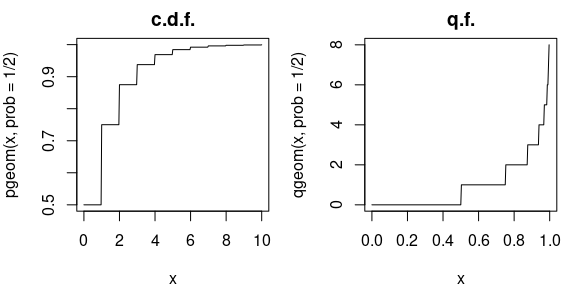Now we study the QME for the geometric distribution. Since we have only one parameter, we choose one probabiliy, $$p=1/2$$. The theoretical median is the following integer $F_X^{-1}(1/2) = \left\lfloor\frac{\log(1/2)}{\log(1-p)}\right\rfloor.$ Note that the theoretical median for a discrete distribution is an integer. Empirically, the median may not be an integer. Indeed for an even length dataset, the empirical median is $q_{n,1/2} = \frac{x_{n/2}^\star + x_{n/2+1}^\star}{2},$ where $$x_{1}^\star<\dots<x_{n}^\star$$ is the sorted sample, which is not an integer value if $$x_{n/2}^\star + x_{n/2+1}^\star$$ is not an even number. However for an odd length dataset, the empirical median is an integer $$q_{n,1/2}=x_{(n+1)/2}^\star$$.

##  0.5
##  0

Therefore, a first issue arises: if the median is not an integer, it is impossible to match exactly the empirical median with the theoretical quantile.

Furthermore, the second issue is the non-uniqueness of the solution. Admitting matching $$q_{n,1/2}$$ is an integer, QME aims to find some $$p$$ such that $\left\lfloor\frac{\log(1/2)}{\log(1-p)}\right\rfloor = q_{n,1/2} \Leftrightarrow q_{n,1/2} \leq \frac{\log(1/2)}{\log(1-p)} < q_{n,1/2} +1.$ Let us plot the squared differences $$(F_X^{-1}(1/2) - q_{n,1/2})^2$$.

##  0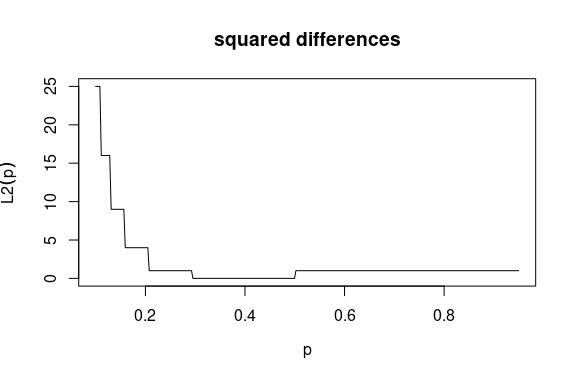Any value between [1/3, 5/9] minimizes the squared differences. Therefore, fitdist() may be sensitive to the chosen initial value with deterministic optimization algorithm.

## initial  value 1.000000
## iter   2 value 0.000000
## iter   2 value 0.000000
## iter   2 value 0.000000
## final  value 0.000000
## converged
## Fitting of the distribution ' geom ' by matching quantiles
## Parameters:
##      estimate
## prob     0.34
## initial  value 144.000000
## iter   1 value 144.000000
## final  value 144.000000
## converged
## Fitting of the distribution ' geom ' by matching quantiles
## Parameters:
##      estimate
## prob     0.05

The solution is to use a stochastic algorithm such as simulated annealing (SANN).

## Fitting of the distribution ' geom ' by matching quantiles
## Parameters:
##      estimate
## prob    0.497
## Fitting of the distribution ' geom ' by matching quantiles
## Parameters:
##      estimate
## prob    0.401

Let us consider the Poisson distribution defined by the following mass probability and the cumulative distribution functions $P(X=k)=\frac{\lambda^k}{k!}\exp(-\lambda),~ F_X(x) = \exp(-\lambda)\sum_{k=0}^{\lfloor x \rfloor}\frac{\lambda^k}{k!},~ x\geq 0.$ The quantile function $$F_X^{-1}(p)=\inf(x, F_X(x)\geq p)$$ simplifies to $F_X^{-1}(p) = i \text{ such that } \sum_{k=0}^{i-1} P(X=k) \leq p < \sum_{k=0}^{i} P(X=k).$ Again, the quantile function is a step function $F_X^{-1}(p) = \left\{ \begin{array}{ll} 0 & \text{if } p < P(X=0) \\ 1 & \text{if } P(X=0) \leq p < P(X=0)+P(X=1) \\ 2 & \text{if } P(X=0)+P(X=1) \leq p < P(X=0)+P(X=1)+P(X=2) \\ \dots \\ i & \text{if } \sum_{k=0}^{i-1} P(X=k) \leq p < \sum_{k=0}^{i} P(X=k) \\ \dots \\ \end{array} \right.$

Again, the squared differences is a step function $$(F_X^{-1}(1/2) - q_{n,1/2})^2$$.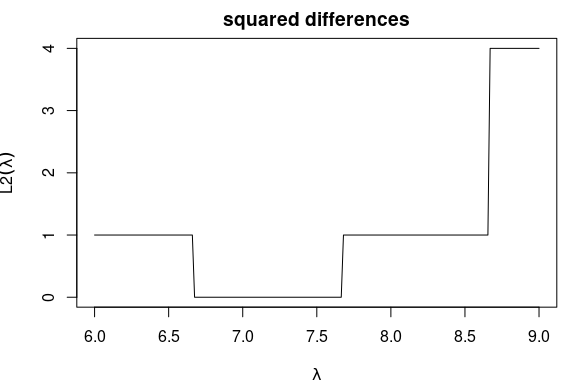Therefore, using fitdist() may be sensitive to the chosen initial value.

## Fitting of the distribution ' pois ' by matching quantiles
## Parameters:
##        estimate
## lambda        2
## Fitting of the distribution ' pois ' by matching quantiles
## Parameters:
##        estimate
## lambda     6.73

4 Questions regarding uncertainty

4.1 Can we compute marginal confidence intervals on parameter estimates from their reported standard error ?

In statistics, deriving marginal confidence intervals on MLE parameter estimates using the approximation of their standard errors (calculated from the hessian) is a quite common procedure. It is based on the wald approximation which stands that when the sample size $$n$$ is sufficiently high, the marginal $$95\%$$ confidence on the ith component $$\theta_i$$ of a model parameter $$\theta$$ estimated by maximum likelihood (estimate denoted $$\hat \theta$$) can be approximated by : $$\hat \theta_i \pm 1.96 \times SE(\hat \theta_i )$$ with $$SE(\hat \theta_i )$$ the ith term of the diagonal of the covariance matrix of the estimates ($$V_{ii}$$). $$V$$ is generally approximated by the inverse of the Fisher information matrix ($$I(\hat \theta)$$). The Fisher information matrix corresponds to the opposite of the hessian matrix evaluated on the MLE estimate. Let us recall that the hessian matrix is defined by $$H_{ij}(y, \theta) = \frac{\partial^2 L(y, \theta)}{\partial \theta_i \partial \theta_j}$$ with $$L(y, \theta)$$ the loglikelihod function for data $$y$$ and parameter $$\theta$$.

Before using this approximation, one must keep in mind that its validity does not only depend on the sample size. It also strongly depends on the data, of the distribution, and also on the parameterization of the distribution. For this reason we recommend potential users of the Wald approximation to compare the results to the ones obtained using the bootstrap procedure (see below) before using this approximation. A look at the loglikelihood contours is also interesting as the Wald approximation assumes elliptical contours. In a more general context, we recommend the use of bootstrap to compute confidence intervals on parameters or on any function of those parameters.

Below you will find two examples, one for which Wald confidence intervals seem correct and one for which they give wrong results, with parameter values even outside their possible range (negative rate bound for the gamma distribution).

##      Median 2.5% 97.5%
## mean   9.41 8.78 10.02
## sd     1.73 1.33  2.15
##      estimate 2.5% 97.5%
## mean     9.41 8.77 10.04
## sd       1.78 1.33  2.22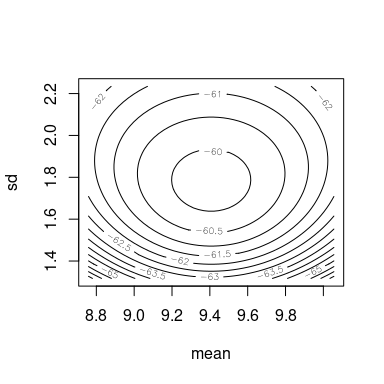##        Median   2.5%   97.5%
## shape  0.0923 0.0636   0.145
## rate  30.0782 9.6306 146.660
##       estimate    2.5%  97.5%
## shape   0.0882  0.0553  0.121
## rate   24.2965 -6.3504 54.944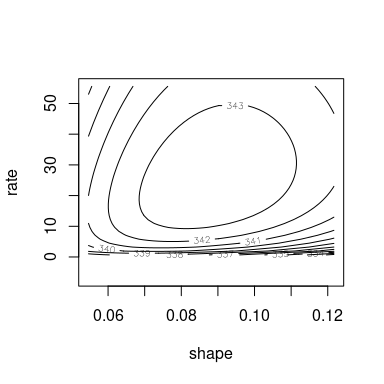4.2 How can we compute confidence intervals on quantiles from the fit of a distribution ?

The quantile() function can be used to calculate any quantile from a fitted distribution when it is called with an object of class fitdist or fitdistcens as the first argument. When called with an object of class bootdist or bootdistcens as the first argument, quantiles are returned accompanied with a confidence interval calculated using the bootstraped sample of parameters. Moreover, you can use the CIcdfplot() function to plot the fitted distribution as a CDF curve surrounded by a band corresponding to pointwise intervals on the quantiles. See an example below on censored data corresponding to 72-hour acute salinity tolerance (LC50values) of rivermarine invertebrates.

## (original) estimated quantiles for each specified probability (censored data)
##          p=0.05
## estimate   1.12
## Median of bootstrap estimates
##          p=0.05
## estimate   1.12
##
## two-sided 95 % CI of each quantile
##        p=0.05
## 2.5 %    1.05
## 97.5 %   1.20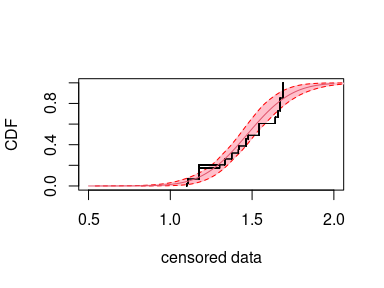4.3 How can we compute confidence intervals on any function of the parameters of the fitted distribution ?

The bootstrap sample of parameter estimates can be used to calculate a bootstrap sample of any variable defined as a function of the parameters of the fitted distribution. From such a bootstrap sample you can easily compute a conidence interval using percentiles. Below is an example that uses the bootstrap sample of parameters from the previous example (FAQ 4.2) to calculate a 95 percent confidence interval on the Potentially Affected Portion (PAF) of species at a given exposure to salinity (fixed to 1.2 in log10 in this example).

##   2.5%  97.5%
## 0.0487 0.1470

For more complex calculations especially to tranfer uncertainty within a quantitative risk assessment, we recommend the use of the package mc2d which aims at making such calculations easy and which gives extensive examples of use of such bootstrap samples of parameters estimated using functions of the package fitdistrplus.

5 How to personalize plots

5.1 Can I personalize the default plot given for an object of class fitdist or fitdistcens?

The default plot given by using the plot() function on an object of class fitdist or fitdistcens is hard to personalize. Indeed this plot was designed only to give a quick overview of the fit, not to be used as a graph in a manuscript or a formal presentation. To personalize some of (or all) the goodness-of-fit plots, you should rather use specific graphical functions, denscomp, cdfcomp, ppcomp, qqcomp or cdfcompcens (see how in the following paragraphs).

5.2 How to personalize goodness-of-fit plots ?

The default plot of an object of class fitdist can be easily reproduced and personalized using denscomp, cdfcomp, ppcomp and qqcomp.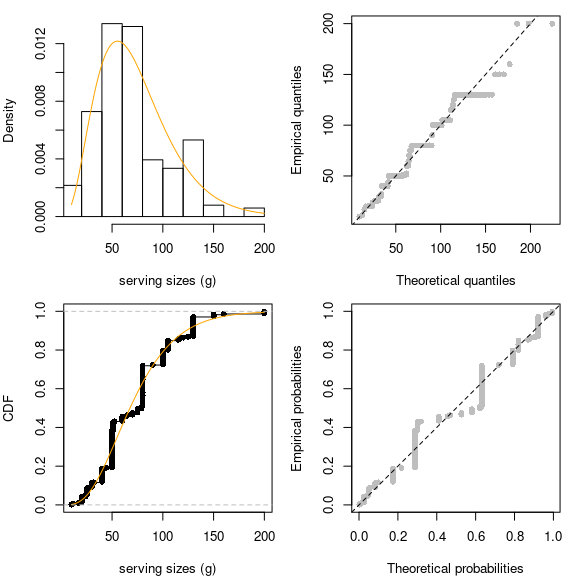In a similar way, the default plot of an object of class fitdistcens can be easily personalized using cdfcompcens.

5.3 Is it possible to obtain ggplot2 plots ?

An argument plotstyle was added to functions denscomp, cdfcomp, ppcomp, qqcompand cdfcompcens, ppcompcens, qqcompcens to enable the generation of plots using the ggplot2 package. This argument by default fixed at graphics must simply be fixed at ggplot for this purpose, as in the following example. In that latter case the graphical functions return a graphic object that can be further personalized using ggplot2 functions.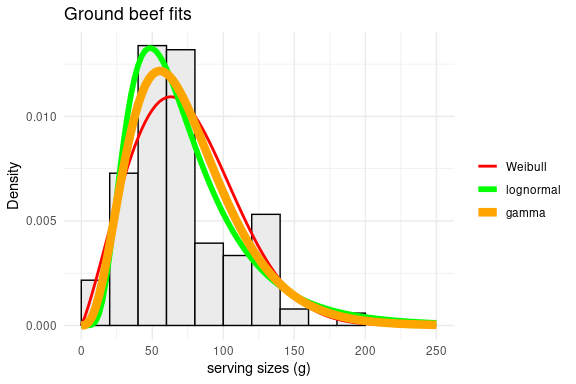5.4 Is it possible to add the names of the observations in a goodness-of-fit plot, e.g. the names of the species in the plot of the Species Sensitivity Distribution (SSD) classically used in ecotoxicology ?

An argument named name.points can be used in functions cdfcomp or CIcdfcomp to pass a label vector for observed points so as to add the names of the points on the left of each point. This option is available only for ECDF goodness-of-fit plots and only for non censored data. This option can be used as below, for example, to name the species in the classical plot of the Species Sensitivity Distributions (SSD) in ecotoxicology.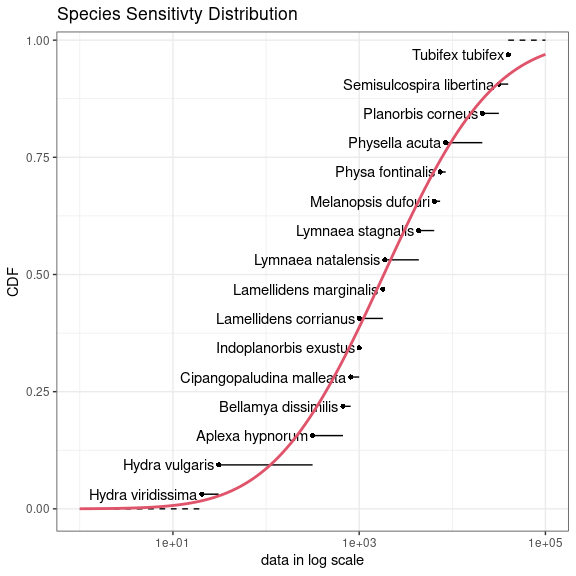6 Questions regarding (left, right and/or interval) censored data

6.1 How to code censored data in fitdistrplus ?

Censored data must be rpresented in the package by a dataframe of two columns respectively named left and right, describing each observed value as an interval. The left column contains either NA for left censored observations, the left bound of the interval for interval censored observations, or the observed value for non-censored observations. The right column contains either NA for right censored observations, the right bound of the interval for interval censored observations, or the observed value for non-censored observations. This type of representation corresponds to the coding names "interval2" in function Surv of the package survival. There is no other way to represent censored data in fitdistrplus but the function Surv2fitdistcens() can be used to help you to format data for use in fitdistcens() from one of the format used in the survival package (see the help page of Surv2fitdistcens()). You have a toy example below.

##   left right
## 1   NA   1.0
## 2  2.0   3.0
## 3  4.0   7.0
## 4  6.0   8.0
## 5  9.7   9.7
## 6 10.0    NA

6.2 How do I prepare the input of fitdistcens() with Surv2fitdistcens()?

Let us consider a classical right-censored dataset for human life: twenty values randomly chosen from the canlifins dataset of CASdatasets package. We refer to the help of Surv2fitdistcens() for other censoring types.

When performing survival analysis, it is very common to use Surv() function from package survival to handle different types of censoring. In order to ease the use of fitdistcens(), a dedicated function Surv2fitdistcens() has been implemented with arguments similar to the ones of Surv().

Let us now fit two simple distributions.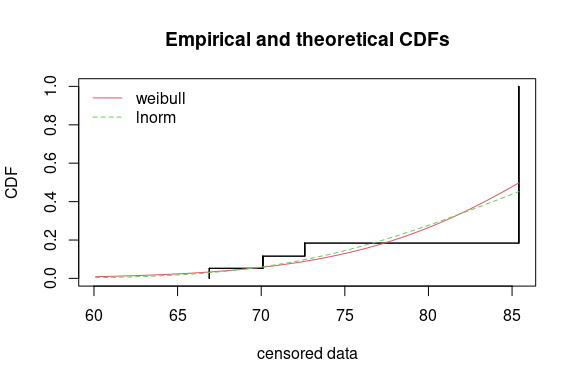6.3 How to represent an empirical distribution from censored data ?

The representation of an empirical distribution from censored data is not a trivial problem. One can simply represent each observation as an interval at an y-value defined by the rank of the observation as done below using function plotdistcens. This representation can be interesting to visualize the raw data, but it remains difficult to correctly order the observations in any case (see the example below on the right using data smokedfish).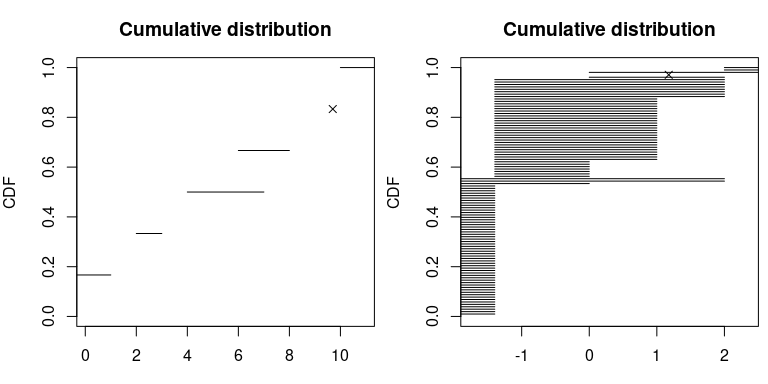Many authors worked on the development of algorithms for non parametric maximum likelihood estimation (NPMLE) of the empirical cumulative distribution function (ECDF) from interval censored data (including left and right censored data that can be considered as interval censored data with one bound at infinity). In old versions of fitdistrplus we used the Turnbull algorithm using calls to functions of the package survival. Even if this Turnbull algorithm is still available in the package, the default plot now uses the function npsurv of the package npsurv. This package provides more performant algorithms developped by Yong Wang (see references cited in the help page of plotdistcens). Due to lack of maintenance of this package we were forced to rewrite their main functions in our package, using another optimization function. The same ECDF plot was also implemented in our using the Turnbull algorithm of survival (see below).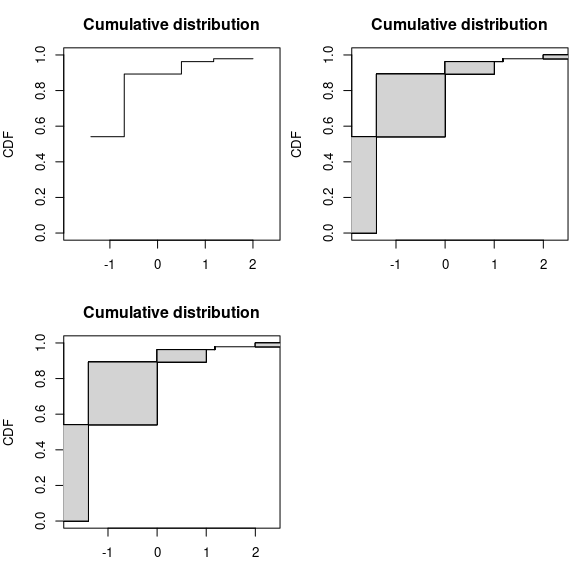As you can see in the above example, the new implementation of NPMLE provides a different type of plot for the ECDF, representing by filled rectangles the zones of non-uniqueness of the NPMLE ECDF. Indeed an NPMLE algorithm generally proceeds in two steps.

1. The first step aims at identifying equivalence classes (also named in the litterture Turnbull intervals or maximal intersection intervals or innermost intervals or maximal cliques of the data). Equivalences classess are points/intervals under which the NPMLE ECDF may change. Equivalence classes have been shown to correspond to regions between a left bound of an interval (named L in the following plot on a the previous toy example) immediately followed by a right bound of an interval (named R in the following plot). An equivalence class may be of null length (for example at each non censored value).

2. The second step aims at assigning a probability mass to each equivalence class, which may be zero on some classes. The NPMLE is unique only up to these equivalence classes and this non uniqueness of the NPMLE ECDF is represented by filled rectangles.

Various NPMLE algorithms are implemented in the packages Icens, interval and npsurv. They are more or less performant and all of them do not enable the handling of other data than survival data, especially with left censored observations.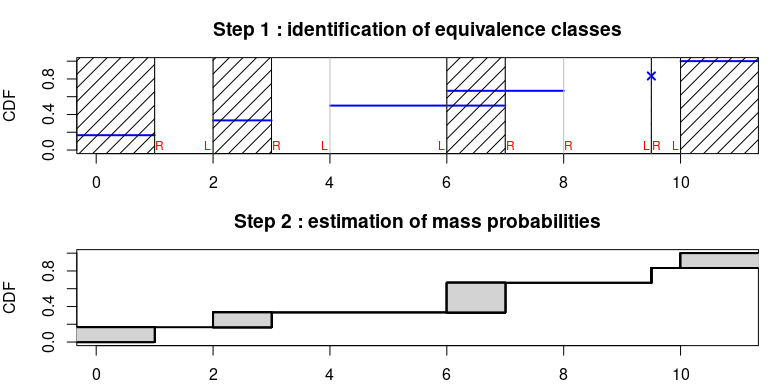6.4 How to assess the goodness-of-fit of a distribution fitted on censored data ?

The only available method in fitdistrplus to fit distributions on censored data is the maximum likelihood estimation (MLE). Once a distribution is fitted using fitdistcens, AIC and BIC values can be found in the summary of the object of class fitdistcens returned by the function. Those values can be used to compare the fit of various distributions on a same dataset. Function gofstat is not yet proposed in our package for fits on censored data but we plan to develop it in the future with the calculation of other goodness-of-fit statistics for censored data.

##  178
##  177

Considering goodness-of-fit plots, the generic plot function of an object of class fitdistcensprovides three plots, one in CDF using the NPMLE ECDF plot (by default using the Wang prepresentation, see previous part for details), a Q-Q plot and a P-P plot simply derived from the Wang plot of the ECDF, with filled rectangles indicating non uniqueness of the NPMLE ECDF.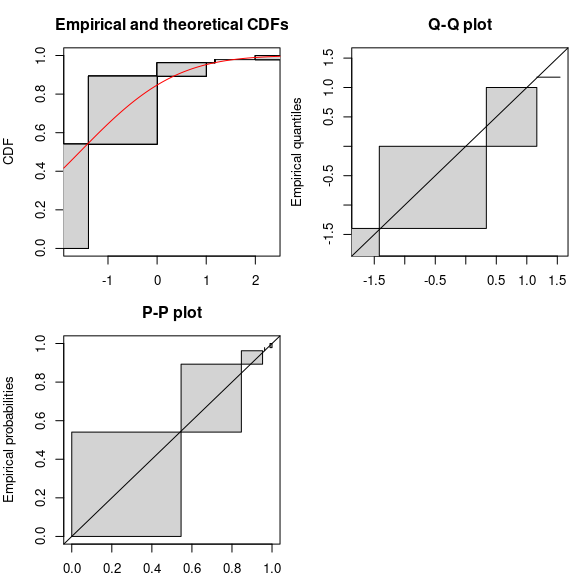Functions cdfcompcens(), qqcompens() and ppcompcens() can be used to individualize and personnalize CDF, Q-Q and P-P goodness-of-fit plots and/or to compare the fit of various distributions on a same dataset.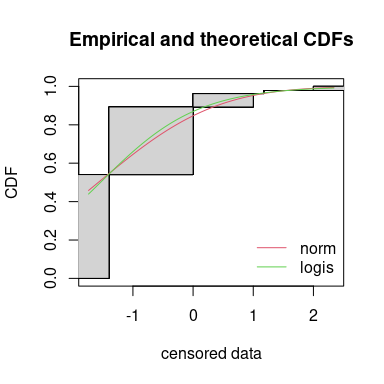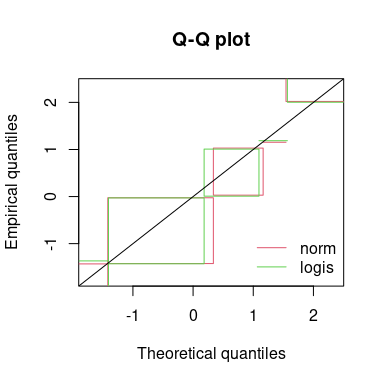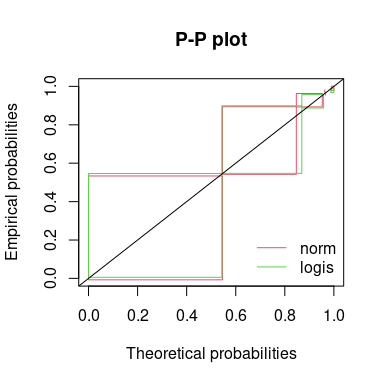Considering Q-Q plots and P-P plots, it may be easier to compare various fits by splitting the plots as below which is done automatically using the plotstyle ggplot in qqcompens() and ppcompcens() but can also be done manually with the plotstyle graphics.